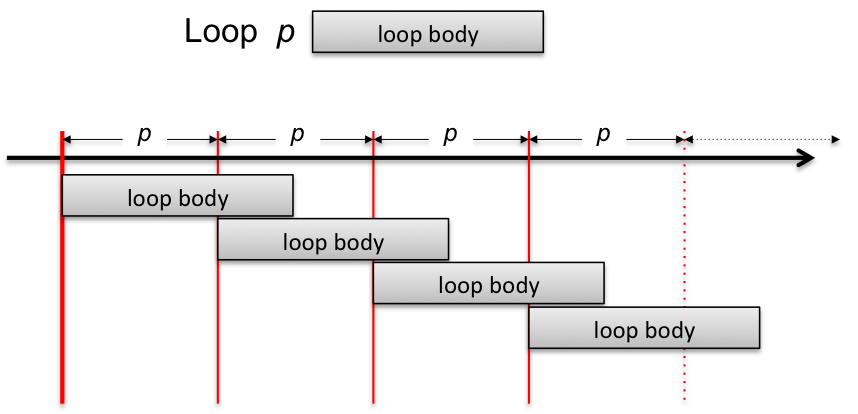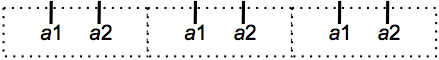## Loop: Sequential Iterations¶

The loop construction

          loop optional_label  period { loop_body }


is similar to a group but instead of being performed once, the actions in the loop body are iterated depending on a period specification giving the time elapsed between two loop iterations:

          Loop L 0.5
{  print $NOW }  will print:  0 0.5 1 1.5 ...  The instances of the loop body are evaluated as independent groups. So, if the period is shorter than the duration of the body of the loop, successive iterations will overlap: $i := 0
Loop L1 1
{
@local $j$j := $i$i := $i+1 print "start"$j
2 print "stop" $j }  will print:  start 0 start 1 stop 0 start 2 stop 1 stop 2  Here, when the body of the loop is instantiated, the global variable $i is copied in the local variable $j: $i can then be updated to count the iteration but $j records the iteration number for a given loop body. The loop period is 1 beat and the duration of the body is 2 beats. So two successive instances of the loop body overlap and their printing are interleaved. Notice that the local variable is local to a loop body instantiation (they are as many $j as concurrent loop bodies).The overlapping of two iterations of the loop body can be avoided, see @exclusive below.

### Loop Period¶

The period of a loop is an expression evaluated at each iteration and is used to schedule the next iteration. So the duration between two iterations can change as the time progress and the iterations are not necessarily periodic.

The period expression is a duration, i.e., it can be absolute or relative.

 $period := 1 Loop$period s
{
print $NOW 0.5 s let$period := $period + 1 }  will print:  0 1 3 6 10 15 ...  When the loop is launched at time 0 second, the body is also launched for the first time and, in parallel, the next iteration is scheduled with the current value of the period (which at this time is 1 second). A 0 is printed. After 0.5 seconds, the variable $period is incremented. At date 1 second, the period for the next iteration is evaluated (to 2) and the second iteration is launched (printing a 1). So after 1+2 seconds, the third iteration takes place and print a 3, etc.

In addition, the period expression can evaluate to a tab (i.e., a vector): in this case, the elements of the vector are the successive periods of the loop. Note that the periods are taken cyclically in the vector. The s or ms qualifier can be used to specify that the tab elements are given in absolute time instead of relative time:

          $p := [100, 200, 400, 800] Loop$p ms
{
print $NOW }  will print:  0 0.1 0.3 0.7 1.5 1.6 ...  ### Stopping a Loop¶ The optional until or while clauses are evaluated at each iteration and eventually stop the loop. For instance, the declarations on the left produce the timing of the action’s firing figured in the right:  let$cpt := 0
loop L 1.5
{
$cpt :=$cpt + 1
0.5 a₁
0.5 a₂
}
until ($cpt >= 3)The previous loop can also be written using a during clause. Logical times corresponds to loop iterations, so:  loop L 1.5 { 0.5 a₁ 0.5 a₂ } during [3#]  is equivalent to the previous loop. Because the loop period is $1.5$, three loop iteration will last $4.5$ beats, so it can be also written:  loop L 1.5 { 0.5 a₁ 0.5 a₂ } during [4.5]  If an end clause is not provided, the loop will continue forever but it can be killed by an explicit abort command:  loop ForEver 1 { print OK } 3.5 abort ForEver  In the case above, OK will only be printed three times. ### Instantaneous Iteration¶ A period of zero (in relative or absolute time) is perfectly legal: all iterations take place in the same instant:  Loop 0ms { print "OK at "$NOW
} during [100#]


will print 100 times OK at xxx at date xxx.

Instantaneous iterations can be used for instance to perform computations on a data-structure (but see also the iteration expression allowed in function definitions).

However, an infinite loop with a zero period implies to perform an infinite number of computations in finite time, which is not possible. For this reason, there is a run-time security: if there is no end clause, the run-time aborts the loop if the number of successive iterations with a period of zero reaches a predefined limit of $10000$.

### Avoiding Overlapping Iterations: @exclusive¶

As mentioned above, two iterations of a loop body may overlap. In some case this is not the intended behavior: the previous iteration must be stopped before starting the new iteration of the loop body. This is achieved by specifying the attribute @exclusive for the loop: with this attribute, the previous iteration and its eventual childs are aborted. For instance, the program

          $i := 0 loop 1 @exclusive { @local$id
$i :=$i + 1
$id :=$i

loop 0.25 { print iteration $id at$NOW }
}

2 antescofo::killall


will print the trace at the left. Without the attribute, the trace is given on the right:

       iteration 1 at 0.0
iteration 1 at 0.25
iteration 1 at 0.5
iteration 1 at 0.75
iteration 2 at 1.0
iteration 2 at 1.25
iteration 2 at 1.5
iteration 2 at 1.75
iteration 2 at 2.0


    iteration 1 at 0.0
iteration 1 at 0.25
iteration 1 at 0.5
iteration 1 at 0.75
iteration 2 at 1.0
iteration 1 at 1.0
iteration 1 at 1.25
iteration 2 at 1.25
iteration 1 at 1.5
iteration 2 at 1.5
iteration 1 at 1.75
iteration 2 at 1.75
iteration 2 at 2.0


Notice that without the attribute, there are two iterations of the loop body that execute the print command at the same date. With the attribute, each iteration of the loop body occurs at disjoint time intervals.

See also the section Priority for the management of actions that take place at the same date.

### Synchronization Attributes of a Loop¶

The loop body is an implicit group and the instances of the loop body are childs of the loop. So, synchronization attributes, like @tempo, defined at the loop level, are inherited by them.